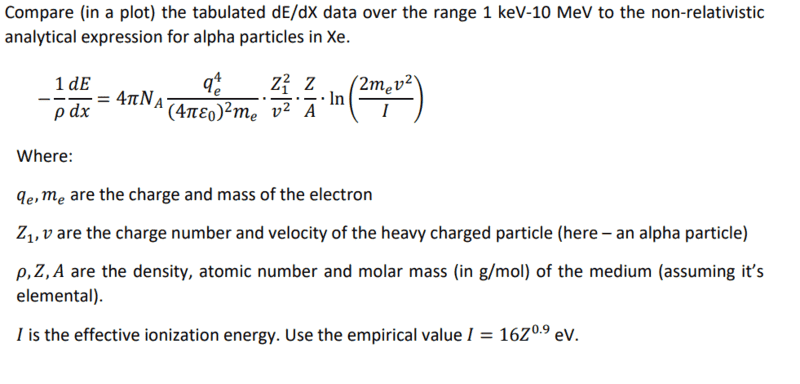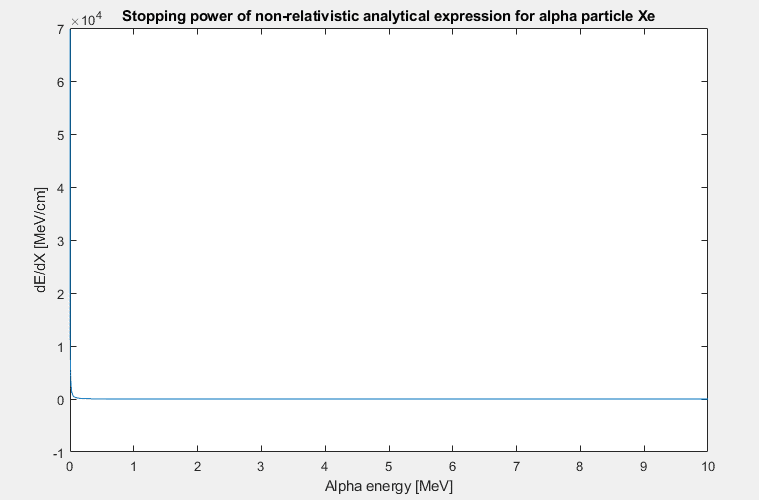Bethe-Bloch calculation

• I
Hi all,

I got an exercise to plot Bethe-Bloch function (ignore the "Compare..." part, it belongs to an exercise above), for alpha particle in Xenon. See the question below:I've been struggling to get a plot that makes sense. I checked my parameters and the units a million times already, but couldn't find where the problem is. On paper, the units I'm left with, after all the conversions, are MeV/cm, which is exactly what I want. But again, I get a weird curve...

Here is the Matlab code I wrote:

Code:
E_dat=[ 0.001    0.002    0.003    0.003    0.004    0.005    0.006    0.007    0.008    0.009    0.010    0.013    0.015    0.018    0.020    0.023    0.025    0.028    0.030    0.035    0.040    0.045    0.050    0.055    0.060    0.065    0.070    0.075    0.080    0.085    0.090    0.095    0.100    0.125    0.150    0.175    0.200    0.225    0.250    0.275    0.300    0.350    0.400    0.450    0.500    0.550    0.600    0.650    0.700    0.750    0.800    0.850    0.900    0.950    1.000    1.250    1.500    1.750    2.000    2.250    2.500    2.750    3.000    3.500    4.000    4.500    5.000    5.500    5.544    6.000    6.500    7.000    7.500    8.000    8.500    9.000    9.500    10.000 ];%MeV

% Constants for Bethe-Bloch equation:
rho = 0.010592; % [g/cm^3] for Xenon in 2 bar and 25 C conditions
qe=1.602e-19; % C
me=9.109e-28; % g
K=9e13; % K=1/4*pi*epsilon0 , units of N*cm^2/C^2
Z1=2;
malpha=6.646e-24; % g
v=sqrt(2*E_dat/malpha); % sqrt(MeV/g)
A=131.293; % g/mol
Z=54;
I=(16*Z^0.9)/1e6; % MeV
first=-rho*4*pi*NA;
second=((qe^4)*(K^2))/me;
third=((Z1^2)./(v.^2));
fourth=Z/A;
fifth=log((2*me*(v.^2))./I);
aaa=first*second*fourth;
bbb=third.*fifth;
dEdX_BB=aaa*bbb*3.9e21; % 3.9e21 is to convert from N^2*cm/MeV to MeV/cm

plot(E_dat,dEdX_BB)
xlabel('Alpha energy [MeV]')
ylabel('dE/dX [MeV/cm]')
title('Stopping power of non-relativistic analytical expression for alpha particle Xe')

I know that the peak shouldn't be that sharp, narrow, high (10^4!) and not that close to zero. Also, the dE/dx trend should be more moderate and to spread much more nicely, than this sharp drop to 0..

Thanks.

Last edited:

mfb
Mentor
You could include your plot here.
Alpha particles at 1 keV will have an extreme energy loss even in a gas.

•vanhees71 and jim mcnamara
hutchphd
Homework Helper
Use a reasonable set of units!!!! Newtons, cm, eV????
What value is the ionization potential?

You could include your plot here.
Alpha particles at 1 keV will have an extreme energy loss even in a gas.
Here's the plot:I know it is not the right plot as the lecturer showed something similar in class and it looked differently...

Use a reasonable set of units!!!! Newtons, cm, eV????
What value is the ionization potential?
There are a lot of variables in this formula. I changed all units so I can end up with MeV/cm. I suppose the problem is indeed relevant to the units, but, as I wrote, I can not find the mistake... hence this post.
The ionization potential is given in eV in the exercise so I turned it to MeV.
Would you mind going over the units and see if I messed up? I've reached to a dead-end...

Last edited:
mfb
Mentor
There is a good reason the energy loss is usually plotted on a logarithmic scale.

•Astronuc and vanhees71
There is a good reason the energy loss is usually plotted on a logarithmic scale.
You are right. However, in this case (as I know what to expect), it should look just fine even on a regular scale.

Astronuc
Staff Emeritus
The curve should look something like - one would have to multiply by atomic density
https://www-nds.iaea.org/stopping/stopping_201410/index.html

Also, not quite as it's alpha particles in air and graphite.
https://www.nist.gov/system/files/documents/2017/04/26/newstar.pdf (see figures A.10 and A.11 respectively), which are somewhat consistent with the plot above.

It would help to plot energy in a log-scale as others suggested.

There should be a peak in S(E) about 0.6 MeV, so something appears to be amiss.

FYI - http://www.srim.org/Citations/Cit 02-54.pdf - but it's not complete.